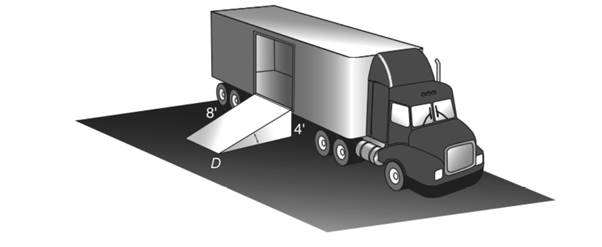Chapter 5.5, Problem 24EElementary Geometry For College St...

7th Edition
Alexander + 2 others
ISBN: 9781337614085

Solutions

Chapter
SectionElementary Geometry For College St...

7th Edition
Alexander + 2 others
ISBN: 9781337614085
Textbook Problem

To unload groceries from a delivery truck at the Piggly Wiggly Market, an 8-ft ramp that rises 4 ft to the door of the trailer is used. What is the measure of the indicated angle ( ∠ D ) ?To determine

To find:

The measure of the indicated angle D. To unload groceries from a delivery truck at the piggy wiggly market, an 8 – ft ramp that rises 4 ft to the door of the trailer is used.

Explanation

Approach:

For a right triangle, for which the measure of the interior angles 30°, 60°, and 90°; if ‘a’ is the length of measure of the shorter leg; opposite to the angle 30°, then the length of the other two sides is given by

Length of the longer leg (opposite to 60°) =a3

Length of the hypotenuse (opposite to 90°)=2a.

In general

Length of the longer leg =3× (Length of the shorter leg)

Length of the hypotenuse =2× (Length of the shorter leg)

Calculation:

Given,

A ramp 8 ft that rises 4 ft.

Clearly, a right ADC is formed with right angle at A, with hypotenuse DC and of two congruent sides AB and AC

Still sussing out bartleby?

Check out a sample textbook solution.

See a sample solution

The Solution to Your Study Problems

Bartleby provides explanations to thousands of textbook problems written by our experts, many with advanced degrees!

Get Started

(916)1/2

Applied Calculus for the Managerial, Life, and Social Sciences: A Brief Approach

Expand each expression in Exercises 122. (x32x2+4)(3x2x+2)

Finite Mathematics and Applied Calculus (MindTap Course List)

Sketch the graph of the equation. 18. y = 2

Single Variable Calculus: Early Transcendentals, Volume I

Factor completely. x2y2

Trigonometry (MindTap Course List)

f(x) dx = F(x) means a) f(x) = F(x) b) f(x) = F(x) c) f(x) = F(b) F(a) d) f(x) = F(x) + C

Study Guide for Stewart's Single Variable Calculus: Early Transcendentals, 8th

True or False: If converges and converges, then converges.

Study Guide for Stewart's Multivariable Calculus, 8th